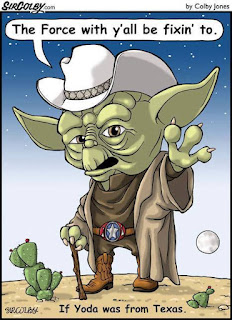## Thursday, September 24, 2009

### The Dallas Cowboys and Math Phobia

If you like The Dallas Cowboys but you don't like math, this article is guaranteed to make you like math. Well, at least you'll see the purpose in being able to solve two simultaneous equations in two unknowns. Wait! Don't stop reading yet. By the time I'm through, it's possible that you might actually understand that some of the stuff you learned and (probably forgot) in Algebra II.

Here's what we're going to do. Given some stuff that's found in most major newspapers, we're going to determine something interesting about the score of The Dallas Cowboys vs. The Carolina Panthers game on Monday, September 28, 2009. If you're reading this after the game, keep reading anyway.

If you look in your paper for the games in the upcoming week, there's usually some information about each upcoming game. For instance, I'm writing this the Thursday before the game and here's what is has:

Favorite: Dallas
Underdog: Carolina
Today's Line: 9
O/U: 47

So what does this mean? The 9 is called 'The Line' and it means that Dallas is expected to win by 9 points. (To be technical, Las Vegas sets 'The Line' so that half the bettors bet on the Cowboys and the other half bet on the Panthers. The rub is that if you bet on the Cowboys, you'll only win if the Cowboys win by more than 9 points). So let's express this with a mathematical equation.

Let X = the Cowboys final score and let Y = the Panthers final score. Then 'The Line' predicts that:

X – Y = 9 (i.e. the Cowboys will win by 9).

Now O/U stands for Over/Under, and that just means that the total score of both the Cowboys and Panthers is expected to be 47 points. So if you bet the 'Over' you'll win your bet if the total score is more than 47 points. The math equation for this is fairly simple as well:

X + Y = 47

Let's put these equations together so we get what mathematicians call two simultaneous equations in two unknowns.

X – Y = 9
X + Y = 47

Now each of these equations separately has lots of solutions. For example the first equation has a solution X = 100 and Y = 91.

The second equation also has a number of solutions, for example, X = 30 and Y = 17.

But what if I asked you for a value for X and a value for Y that satisfy BOTH of the equations. If you found these values, you just solved two simultaneous equations in two unknowns. There are math techniques that allow one to solve these, but you can try to do so by guessing.

Did you get a solution? Not to worry, because here it is:

X = 28 and Y = 19. Note that their difference is 9 and their sum is 47, just what the equations called for.

Now you're going to ask, 'Who cares, and what does this mean anyway?'.

What this means is that the most likely score of the game is:

Cowboys 28
Panthers 19

Don't get me wrong. It is NOT likely that this will be the final score; it's just that this is the most likely score. So if you go to Vegas and had to bet on a final score, this score would have the best chance of winning.

Now don't pick up the phone and call your bookie. Vegas in the long run always wins, so you'll just be throwing your money away. I'm not much of a betting man myself, except that I irrationally spend \$1 per week on a Texas Lottery ticket. I do play an occasional game of Texas Hold 'Em with my nephew and his friends, but I always win, so that really isn't gambling.

OK, back to the main point. By using what's in the paper, and being able to solve two simultaneous equations in two unknowns, you can predict the final score of any football game. How cool is that?

Now repeat after me, "Math = fun."

___________________________________

Tim Farage is a Senior Lecturer in the Computer Science Department at The University of Texas at Dallas. You are welcome to comment upon this blog entry and/or to contact him at tfarage@hotmail.com.
_____________________________________

### No, Aliens Have Not Been To Earth

We're still not sure about these facts, but our Milky Way Galaxy contains around a trillion stars, and there are estimated to be a trill...